# Graphing Derivatives and L'Hopital's Rule: Help and Review Chapter Exam

Exam Instructions:

Choose your answers to the questions and click 'Next' to see the next set of questions. You can skip questions if you would like and come back to them later with the yellow "Go To First Skipped Question" button. When you have completed the practice exam, a green submit button will appear. Click it to see your results. Good luck!

### Page 1

#### Question 1 1. Find the following limit: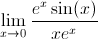#### Question 3 3. Of the three limits shown below, which can be evaluated using L'Hopital's Rule?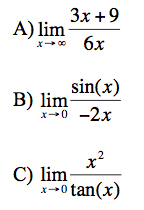#### Question 4 4. Which of the following graphs is the derivative of the graph below?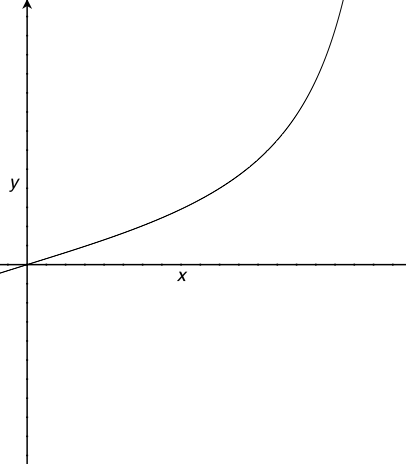#### Question 5 5. Given the graph of f'(x) below, what can you say about f(x) between x=0 and x=4?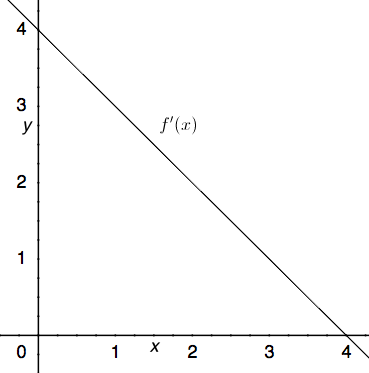### Page 2

#### Question 7 7. Consider the function h(x) below. At x = 1 is this function concave up, concave down, or an inflection point?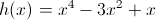#### Question 8 8. Which of the following is a local minimum?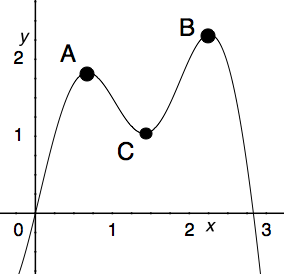#### Question 10 10. Which of the following BEST describes point C?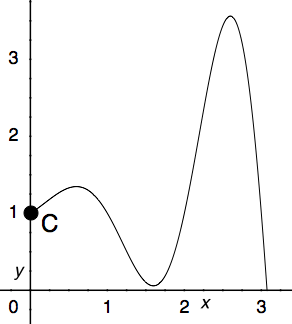### Page 3

#### Question 11 11. The derivative of the following graph is _____ at point C.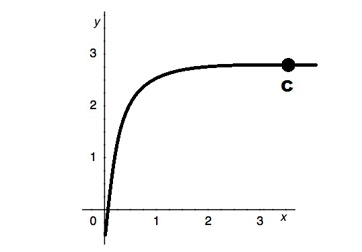#### Question 13 13. What is the equation of the line tangent to f(x) at the point x=0?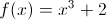#### Question 14 14. What is the equation of the line normal to f(x) at the point x=1?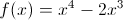#### Question 15 15. Evaluate the limit of the following: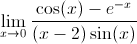### Page 4

#### Question 16 16. What is the slope of the tangent to r(s) at s = 0?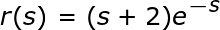#### Question 17 17. What is the slope of the line normal to f(x) at x=1?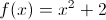#### Question 18 18. The graph shown is an example of a function that: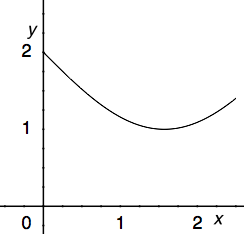#### Question 19 19. Evaluate the limit shown below: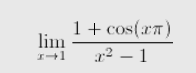### Page 5

#### Question 21 21. Consider the function g(x) below. At x = 0 is this function concave up, concave down, or an inflection point?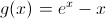#### Question 22 22. Given the graph of f'(x) below, what could f(x) look like in the region between x=0 and x=4?#### Question 23 23. Given the graph of f'(x) below, what can you say about f(x)?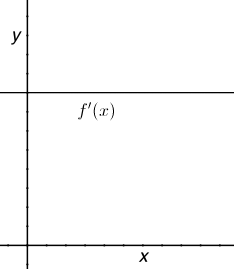#### Question 24 24. Evaluate the limit shown below: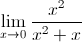#### Question 25 25. Given the graph of f '(x) below, what can you say about f(x) between x=0 and x=4?### Page 6

#### Question 26 26. Consider the function f(x) below. At x = 2 is this function concave up, concave down, or an inflection point?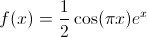#### Question 27 27. Consider the function f(x) below. At x = 1 is this function concave up, concave down, or an inflection point?#### Question 28 28. Which could be the graph of the derivative of the function below?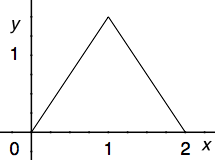#### Question 30 30. Which of the following BEST describes point B?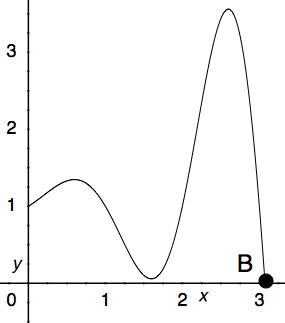#### Graphing Derivatives and L'Hopital's Rule: Help and Review Chapter Exam Instructions

Choose your answers to the questions and click 'Next' to see the next set of questions. You can skip questions if you would like and come back to them later with the yellow "Go To First Skipped Question" button. When you have completed the practice exam, a green submit button will appear. Click it to see your results. Good luck!

Support